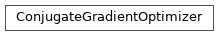# garage.torch.optimizers¶

PyTorch optimizers.

class ConjugateGradientOptimizer(params, max_constraint_value, cg_iters=10, max_backtracks=15, backtrack_ratio=0.8, hvp_reg_coeff=1e-05, accept_violation=False)

Bases: torch.optim.OptimizerPerforms constrained optimization via backtracking line search.

The search direction is computed using a conjugate gradient algorithm, which gives x = A^{-1}g, where A is a second order approximation of the constraint and g is the gradient of the loss function.

Parameters
• params (iterable) – Iterable of parameters to optimize.

• max_constraint_value (float) – Maximum constraint value.

• cg_iters (int) – The number of CG iterations used to calculate A^-1 g

• max_backtracks (int) – Max number of iterations for backtrack linesearch.

• backtrack_ratio (float) – backtrack ratio for backtracking line search.

• hvp_reg_coeff (float) – A small value so that A -> A + reg*I. It is used by Hessian Vector Product calculation.

• accept_violation (bool) – whether to accept the descent step if it violates the line search condition after exhausting all backtracking budgets.

step(self, f_loss, f_constraint)

Take an optimization step.

Parameters
• f_loss (callable) – Function to compute the loss.

• f_constraint (callable) – Function to compute the constraint value.

property state(self)

dict: The hyper-parameters of the optimizer.

class DifferentiableSGD(module, lr=0.001)

DifferentiableSGD performs the same optimization step as SGD, but instead of updating parameters in-place, it saves updated parameters in new tensors, so that the gradient of functions of new parameters can flow back to the pre-updated parameters.

Parameters
• module (torch.nn.module) – A torch module whose parameters needs to be optimized.

• lr (float) – Learning rate of stochastic gradient descent.

step(self)

Take an optimization step.

zero_grad(self)

Sets gradients of all model parameters to zero.

class OptimizerWrapper(optimizer, module, max_optimization_epochs=1, minibatch_size=None)

A wrapper class to handle torch.optim.optimizer.

Parameters
• optimizer (Union[type, tuple[type, dict]]) – Type of optimizer for policy. This can be an optimizer type such as torch.optim.Adam or a tuple of type and dictionary, where dictionary contains arguments to initialize the optimizer. e.g. (torch.optim.Adam, {‘lr’ : 1e-3}) Sample strategy to be used when sampling a new task.

• module (torch.nn.Module) – Module to be optimized.

• max_optimization_epochs (int) – Maximum number of epochs for update.

• minibatch_size (int) – Batch size for optimization.

get_minibatch(self, *inputs)

Yields a batch of inputs.

Notes: P is the size of minibatch (self._minibatch_size)

Parameters

*inputs (list[torch.Tensor]) – A list of inputs. Each input has shape $$(N \dot [T], *)$$.

Yields

list[torch.Tensor]

A list batch of inputs. Each batch has shape

$$(P, *)$$.

zero_grad(self)

Clears the gradients of all optimized torch.Tensor s.

step(self, **closure)

Performs a single optimization step.

Parameters

**closure (callable, optional) – A closure that reevaluates the model and returns the loss.